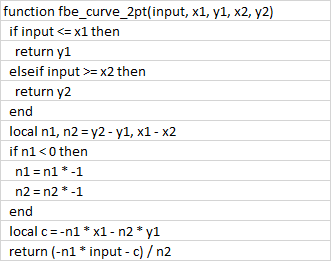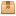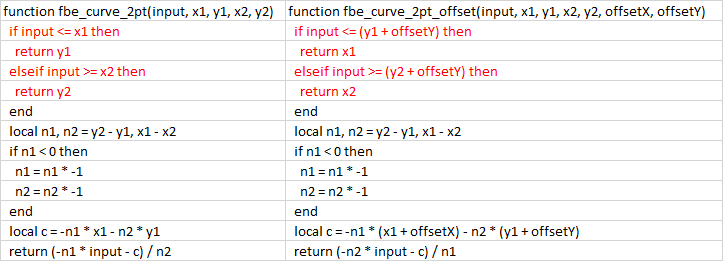This forum uses cookies This forum makes use of cookies to store your login information if you are registered, and your last visit if you are not. Cookies are small text documents stored on your computer; the cookies set by this forum can only be used on this website and pose no security risk. Cookies on this forum also track the specific topics you have read and when you last read them. Please confirm whether you accept or reject these cookies being set.

 FB EditorKoBraJunior Member Posts: 44 Threads: 9 Joined: Apr 2018 Reputation: 1 12.01.2021, 10:38 I found in the latest release 20201210 FB Editor 2.0 the function to calculate a sliding setpoint with or without offset. I tried it but there seems a bug in the one with offset:Does somebody also know why the settings are only available as value and not object? For installers i normally make the X and Y value programmable. For end user normally only the Offset on Y is a setting (but this is also only available as value).Daniel.Senior Member Posts: 2491 Threads: 15 Joined: Aug 2017 Reputation: 96 12.01.2021, 10:50 This is defined in the function which defines this block. Here are all the function https://forum.logicmachine.net/showthrea...49#pid4849. You can import them to user libraries and modify. This is quite old one and not sure if this is same like in the current FB. ------------------------------ Ctrl+F5KoBraJunior Member Posts: 44 Threads: 9 Joined: Apr 2018 Reputation: 1 12.01.2021, 12:20 (This post was last modified: 12.01.2021, 12:22 by KoBra.) Nope i checked and tested this one has the bug in both. So it seems the curve with 2 points function is updated sometime ago. with the data above the outcome for both is now -10Daniel.Senior Member Posts: 2491 Threads: 15 Joined: Aug 2017 Reputation: 96 12.01.2021, 12:23 I will try to get updated libs but it was done buy external dev so it might take some time. ------------------------------ Ctrl+F5KoBraJunior Member Posts: 44 Threads: 9 Joined: Apr 2018 Reputation: 1 13.01.2021, 09:15 I reverse engineered it and used some excel to test the calculations and the one below works. --- [Function] fbe_curve_2pt --- Curve function with 2 points --- [Comment] --- Depending on a straight line defined by 2 points, this module supplies a y-value for an x-value. --- The first point of the straight line is defined by x1 and y1. --- The second point of the straight line is defined by x2 and y2. --- If x is less than x1, then y=y1. --- If x is greater than x2, then y=y2. --- Only strictly monotone rising or falling functions can be realised. This means x1 < x2. --- [Input] --- Input [value, object, storage] --- X1 [value, object, storage] --- Y1 [value, object, storage] --- X2 [value, object, storage] --- Y2 [value, object, storage] --- [Output] --- out - Output [object, storage] function fbe_curve_2pt(input, x1, y1, x2, y2) if input <= x1 then return y1 elseif input >= x2 then return y2 end local n1, n2 = y2 - y1, x1 - x2 if n1 < 0 then n1 = n1 * -1 n2 = n2 * -1 end local c = n1 / n2 return y2 + (x2 - input) * c end --- [Function] fbe_curve_2pt_offset --- Curve function with 2 points and offset --- [Comment] --- Depending on straight lines defined by 2 points, this module supplies an x-value for a y-value. --- The first point of the straight line is defined by x1 and y1. --- The second point of the straight line is defined by x2 and y2. --- The x and the y-axis can be shifted by the respective offset. --- If x is less than (x1 offset x), then y=y1. --- If x is greater than (x2 offset x), then y=y2. --- [Input] --- Input [object, storage] --- X1 [value, object, storage] --- Y1 [value, object, storage] --- X2 [value, object, storage] --- Y2 [value, object, storage] --- Offset X [value, object, storage] --- Offset Y [value, object, storage] --- [Output] --- out - Output [object, storage] function fbe_curve_2pt_offset(input, x1, y1, x2, y2, offsetX, offsetY) if input <= (x1 + offsetX) then return (y1 + offsetY) elseif input >= (x2 + offsetX) then return (y2+ offsetY) end local n1, n2 = y2 - y1, x1 - x2 if n1 < 0 then n1 = n1 * -1 n2 = n2 * -1 end local c = n1 / n2 return (y2 + offsetY) + ((x2 + offsetX) - input) * c endDaniel.Senior Member Posts: 2491 Threads: 15 Joined: Aug 2017 Reputation: 96 14.01.2021, 10:46 Libraries from latest FB Attached FilesAlerts.tar.gz (Size: 1.21 KB / Downloads: 5)Comparator.tar.gz (Size: 1.93 KB / Downloads: 4)Control.tar.gz (Size: 7.88 KB / Downloads: 5)Converter.tar.gz (Size: 4.84 KB / Downloads: 2)DALI.tar.gz (Size: 921 bytes / Downloads: 2)Filter.tar.gz (Size: 743 bytes / Downloads: 2)Gate.tar.gz (Size: 1.79 KB / Downloads: 2)Math_advanced.tar.gz (Size: 4.3 KB / Downloads: 4)Math_basic.tar.gz (Size: 1.83 KB / Downloads: 2)Select_switch.tar.gz (Size: 1.66 KB / Downloads: 2)String.tar.gz (Size: 2.95 KB / Downloads: 2)Time.tar.gz (Size: 6.06 KB / Downloads: 3) ------------------------------ Ctrl+F5KoBraJunior Member Posts: 44 Threads: 9 Joined: Apr 2018 Reputation: 1 14.01.2021, 11:44 (14.01.2021, 10:46)Daniel. Wrote: Libraries from latest FBI found the problem, without the offset the X is the input and the Y the output. In the one with offset the Y is the input and the X the output...KoBraJunior Member Posts: 44 Threads: 9 Joined: Apr 2018 Reputation: 1 14.01.2021, 12:53 The adapted script, where in both versions the X is input (outdoor air temperature for example) and the calculated Y is output. --- [Function] fbe_curve_2pt --- Curve function with 2 points --- [Comment] --- Depending on a straight line defined by 2 points, this module supplies a y-value for an x-value. --- The first point of the straight line is defined by x1 and y1. --- The second point of the straight line is defined by x2 and y2. --- If x is less than x1, then y=y1. --- If x is greater than x2, then y=y2. --- Only strictly monotone rising or falling functions can be realised. This means x1 < x2. --- [Input] --- Input [value, object, storage] --- X1 [value, object, storage] --- Y1 [value, object, storage] --- X2 [value, object, storage] --- Y2 [value, object, storage] --- [Output] --- out - Output [object, storage] function fbe_curve_2pt(input, x1, y1, x2, y2) if input <= x1 then return y1 elseif input >= x2 then return y2 end local n1, n2 = y2 - y1, x1 - x2 if n1 < 0 then n1 = n1 * -1 n2 = n2 * -1 end local c = -n1 * x1 - n2 * y1 return (-n1 * input - c) / n2 end --- [Function] fbe_curve_2pt_offset --- Curve function with 2 points and offset --- [Comment] --- Depending on straight lines defined by 2 points, this module supplies an x-value for a y-value. --- The first point of the straight line is defined by x1 and y1. --- The second point of the straight line is defined by x2 and y2. --- The x and the y-axis can be shifted by the respective offset. --- If x is less than (x1 offset x), then y=y1. --- If x is greater than (x2 offset x), then y=y2. --- [Input] --- Input [object, storage] --- X1 [value, object, storage] --- Y1 [value, object, storage] --- X2 [value, object, storage] --- Y2 [value, object, storage] --- Offset X [value, object, storage] --- Offset Y [value, object, storage] --- [Output] --- out - Output [object, storage] function fbe_curve_2pt_offset(input, x1, y1, x2, y2, offsetX, offsetY) if input <= (x1 + offsetX) then return y1 elseif input >= (x2 + offsetX) then return y2 end local n1, n2 = y2 - y1, x1 - x2 if n1 < 0 then n1 = n1 * -1 n2 = n2 * -1 end local c = -n1 * (x1 + offsetX) - n2 * (y1 + offsetY) return (-n1 * input - c) / n2 end « Next Oldest | Next Newest »

Forum Jump: